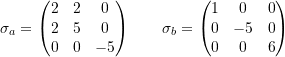### Python Lab Tutorials: Lab 1

1. Write a function in Python whose inputs are another function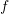and two valuesand. If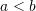, the output of the function should be the vector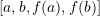, otherwise, the function should return the message: “Please ensure thatis less than“. Show that this function works properly for different cases.
2. Use a “for” loop to create a function whose input is a vector of numbers. The function should check for the first occurrence of a number whose absolute value is less than 0.0005. The function should output the index number and that particular value. For example, if the input is the vector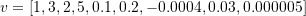, then the output should be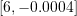. If none of the absolute values of the components of the vector is less than 0.0005, the function should output: “N/A”. Repeat the problem using a “while” loop.
3. The von Mises stress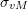(or sometimes called equivalent stress) gives a positive measure of the state of stress given by the symmetric stress matrix: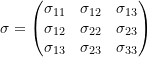is defined as: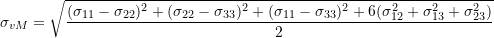Create a function in Python whose argument is a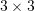matrix and whose output is the von Mises stress. Use this function to show that the equivalent stress is the same for the following stress matrices (units of MPa):